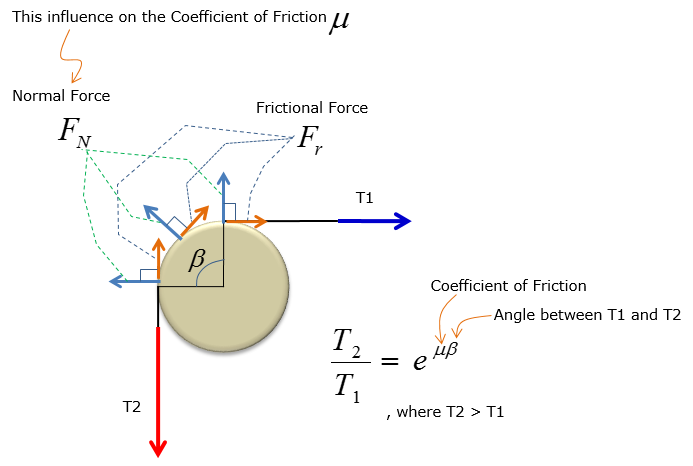Mechanical Engineering - Solid Mechanics                                 Home : www.sharetechnote.com Statics and Friction : Pulley   In Solid Mechanics 1, you have to account for friction and angle to calculate the force exerted by the pulley on a rigid beam at equilibrium. The pulley analysis is always based on the following FBD(Free Body Diagram) :Important notes for the static equilibrium pulley analysis: The angle between T1 and T2 range from 0 to 2 PI radians The equation is based on setting T2 as the force counteracting T1 and Fr, where T2 > T1 in any pulley figurations